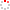#CodeStepByStep## nCopies

Language/Type: JavaScript arrays traversals

Write a function named `nCopies` that accepts an array of integers a as an argument and returns a new array a2, with each element value n from a replaced by n consecutive copies of the value n at the same relative location in the array.

For example, if an array named `a` stores the following element values:

`[3, 5, 0, 2, 2, -7, 0, 4]`

Then the call of `let a2 = nCopies(a)` should return a new array `a2` containing the following elements. The idea is that the value 3 was replaced by three 3s; the 5 was replaced by five 5s; and so on.

`[3, 3, 3, 5, 5, 5, 5, 5, 2, 2, 2, 2, 4, 4, 4, 4]`

Any element whose value is 0 or negative should not be kept in the returned array (as with 0 and -7 above).

The array you return must have a length that is exactly long enough to fit its elements. For example, the result array `a2` above contains 3 + 5 + 2 + 2 + 4 = 16 total elements, so the returned array's length must be exactly 16 in that case. You may assume that the array passed is non-null and that it contains only integers.

Constraints:

• In solving this problem, you must create a single new array to be returned, but aside from that, do not create any other data structures such as temporary arrays or strings.
• You may use as many simple variables (such as numbers) as you like.

Type your JavaScript solution code here:

This is a function exercise. Write a JavaScript function as described. Do not write a complete program; just the function(s) above.